Hello everyone. Today I found some time to complete my review on descriptive statistics, and as always, I decided to involve you in this study process. How? Well, by writing my notes here on the blog in order to share with you what I learn and be corrected by those who know more than me, if necessary.

In the last article, we talked about descriptive statistics. Remember? It's one branch of statistics, along with the study of probability and inferential statistics. In this article, I would like to add a concept that, in my opinion, strongly falls into the category of descriptive statistics. I'm referring to the presentation of data using graphs. Try to imagine yourself for a moment in the example we discussed in the previous article, where we worked as statisticians for a large agricultural company. We tested an agricultural product recommended by a consultant on 20 tomato plants and compared the tomato yield with that of 20 untreated plants (control). After collecting and analyzing the data, we presented the results to our boss using summary statistics such as the mean and standard deviation.

Often, relying solely on summary statistics may not be adequate for presenting study results. This is especially true when you have to communicate the results to an audience that is not familiar with statistics, for whom statistical indicators may be insignificant. It is necessary to capture their attention by focusing on what is truly important. Beautiful plots are needed to help them understand the obtained results.

Presenting results through graphs is not easy at all. I find myself searching on Google to understand which type of plot is most suitable for presenting a certain type of results. It can be said that there is a true discipline in this regard, known as "Data storytelling," which is discussed in various popular books and more.

So, let's see what are the main types of plots that can be used to present your results or, in general, to visualize our data.

### Pie charts:

Pie charts allow you to visualize the values of a variable. In fact, in the "pie," each "slice" corresponds to a value of the variable, and its size is determined by the absolute frequency and the total size of the sample, as shown in the image below: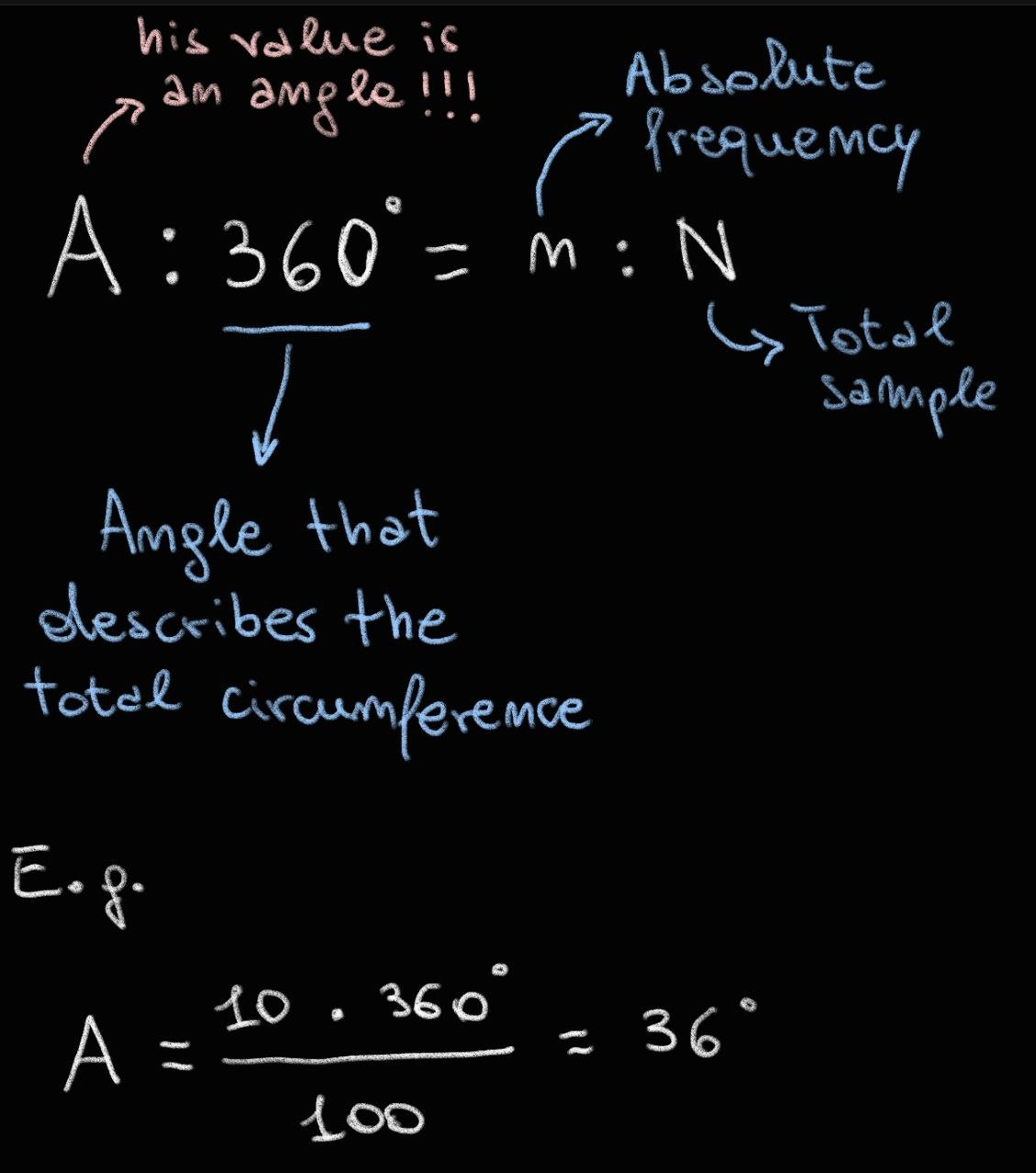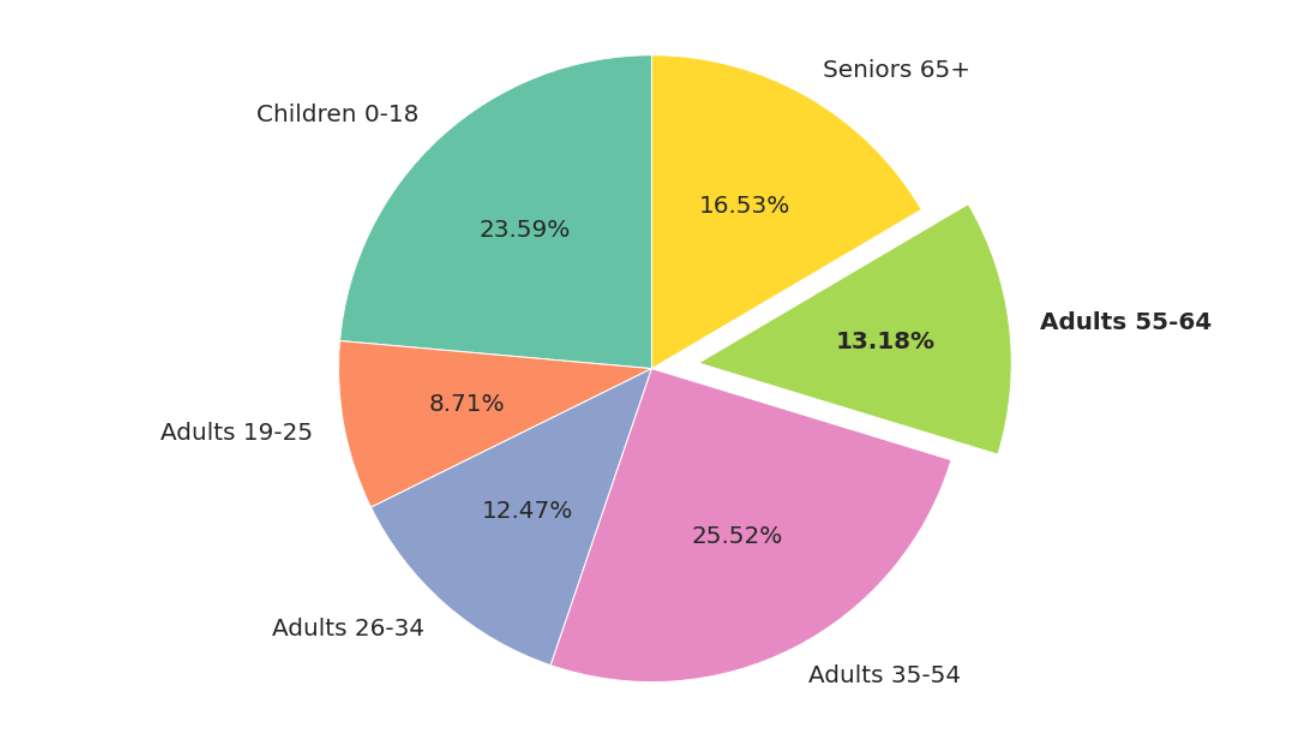Let's see how to create a pie chart in R and python:

In R:

``````# Load the iris dataset
data(iris)
# Calculate the proportions of each species
species_counts <- table(iris\$Species)
species_proportions <- prop.table(species_counts)
# Create a pie chart
pie(species_proportions, labels = paste0(names(species_proportions), " ", round(species_proportions, digits=2)), main = "Pie Chart of Iris Species")
``````

In Python:

``````import pandas as pd
import matplotlib.pyplot as plt

# Calculate the proportions of each species
species_counts = iris['species'].value_counts()
species_proportions = species_counts / species_counts.sum()
# Create a pie chart
plt.pie(species_proportions, labels=species_proportions.index,
autopct=lambda p: f'{p:.2f}%')
plt.title('Pie Chart of Iris Species')
plt.show()``````

### The Bar Plots:

In this type of graph, the values of the variable under examination are placed on the X axis and the respective absolute frequencies on the Y axis.Let's see how to create a Bar Plot in R and python:

In R:

``````# Load the iris dataset
data(iris)

ggplot(iris) +
aes(
x = Species,
fill = Species,
colour = Species,
weight = Petal.Length
) +
geom_bar() +
scale_fill_hue(direction = 1) +
scale_color_hue(direction = 1) +
theme_minimal()``````

In Python:

``````import pandas as pd
from matplotlib import pyplot as plt

df = pd.DataFrame(data)

species = df['species']
petal_length = df['petal_length']

# Figure Size
fig = plt.figure(figsize =(10, 7))

# Horizontal Bar Plot
plt.bar(species, petal_length)

# Show Plot
plt.show()``````

There are several variations of bar plots, and covering all of them in this article would be excessive, so I will provide you with some links at the end of the post for further exploration. However, it's important to know that when we are working with a variable that somehow describes a temporal order, you can connect the "heads" of the bars with a line to highlight the trend over time of the recorded absolute frequency values. Remember that when measuring the values of the same variable over time on the same statistical unit or sample, we talk about time series.
Take a look at the example in the photo below to better understand: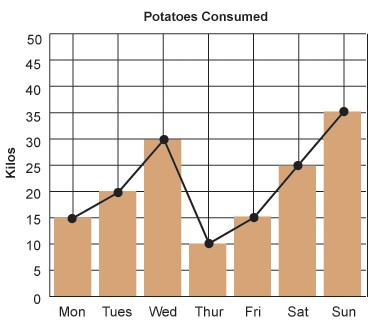### The Box Plot:

When dealing with a series of continuous data and wanting to represent the position and variability indices of these data, it is appropriate to use a box plot or whisker plot. In the image below, you can see all the elements that characterize a box plot: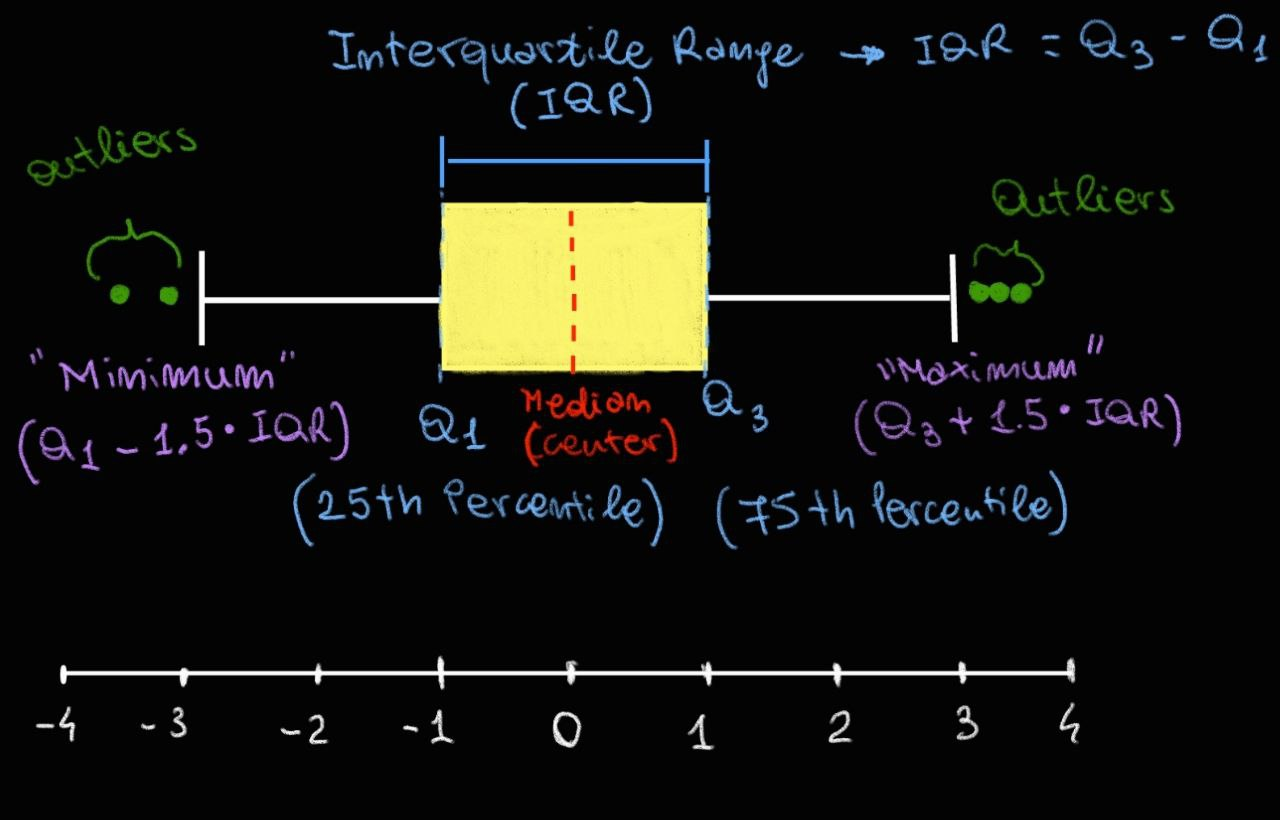Furthermore, it is easy to compare different data series using box plots.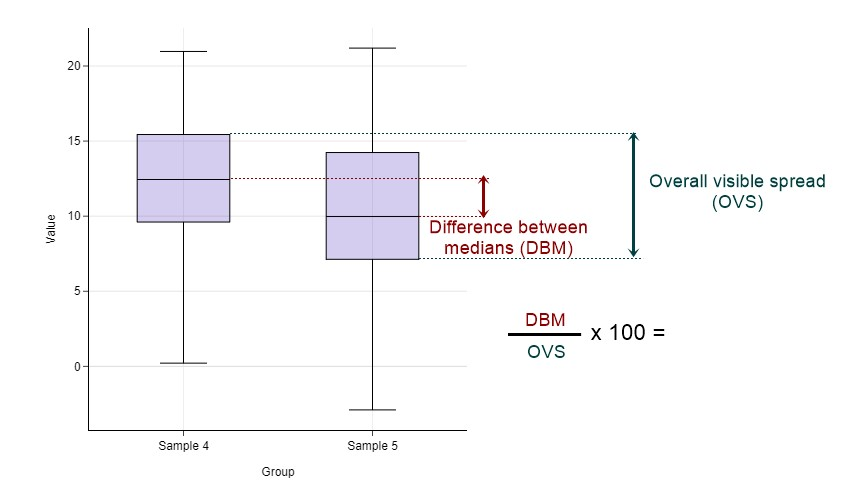Let's see how to create a Box Plot in R and python:

In R:

``````# Load the iris dataset
data(iris)

ggplot(iris) +
aes(x = Petal.Length, y = Species, fill = Species) +
geom_boxplot() +
scale_fill_hue(direction = 1) +
theme_minimal()``````

In Python:

``````import seaborn as sns
import matplotlib.pyplot as plt

# Load the iris dataset (assuming you have the dataset available)

# Create the boxplot
sns.set(style='whitegrid')
plt.figure(figsize=(8, 6))
sns.boxplot(x='petal_length', y='species', data=iris, palette='husl')

# Show the plot
plt.show()``````

These are just a few examples of graphs among those that can be used. Covering them all one by one would be pure madness, but I hope that with these examples, you have an idea of how graphs can effectively, quickly, and non-dispersively convey data even to a non-expert audience. Using the right graph for each occasion and type of result is certainly not easy, but with experience, it will become easier, or at least that's what they told me!

Well, with this article, we have concluded the overview of descriptive statistics. In the next article, we will start discussing another important branch of statistics, namely "Probability Theory." As always, I invite you to leave a comment for clarifications and corrections.

Goodbye and see you soon.

Some references: